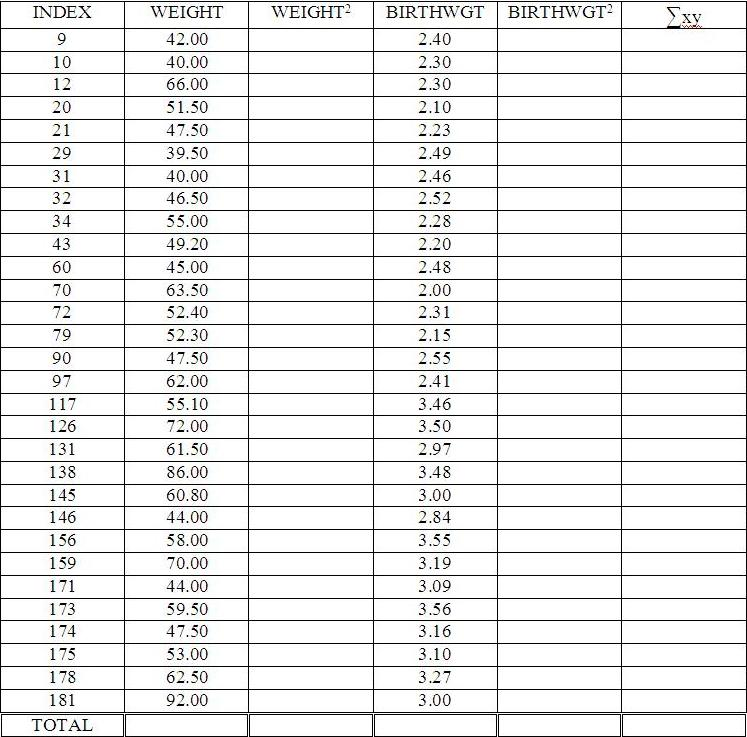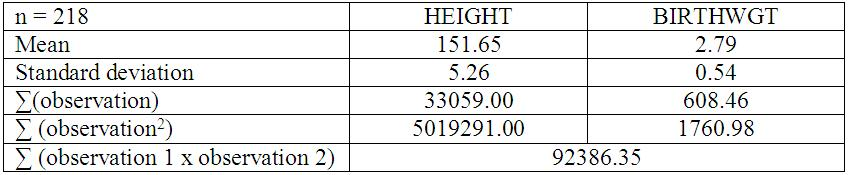# FF2613 - Correlation & Regression

Correlation & Regression Practical
This is a required question

## A study to identify factors that can cause small for gestational age (SGA) babies was conducted. The researcher was trying to prove whether there was any association between the mothers’ first trimester weight (WEIGHT) and the child’s birth weight (BIRTHWGT). Assume that data for both WEIGHT and BIRTHWGT were normally distributed.This is a required question
This is a required question
This is a required question
This is a required question
This is a required question
This is a required question

## A case-control study to identify factors that can cause small for gestational age - SGA was conducted. Among the factors studied were whether there is an association between the mothers' height in cm (HEIGHT) and the child's birth weight in kilogram (BIRTHWGT). Data normally distributed. Conduct the appropriate statistical test.This is a required question
This is a required question
This is a required question